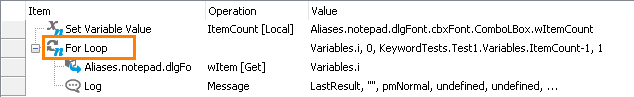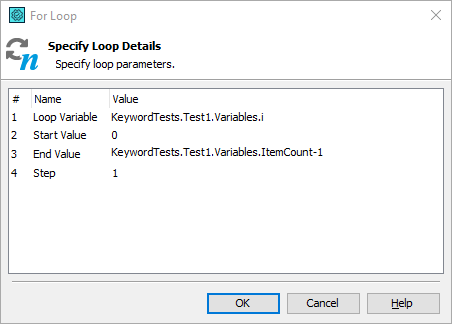### Description

The For Loop operation executes child operations for the specified number of times in a loop.### Operation Result

The operation does not return any value.

### Scripting Analogue

The operation is analogue to the `for` statements of programming languages like C++, Visual Basic, JavaScript or Delphi.

### Child Operations

The operation can have any other operation as a child (or children).

For information on how to make an operation a child of another operation, see the description of the Keyword Test editor’s Test Steps page.

### Operation Parameters

The operation has the following parameters:

Loop Variable

Specifies the name of a variable that will be used as the loop variable. It is used to determine the number of loops (see Remarks below). If you do not specify the loop variable, it will be created automatically when the loop starts and deleted when the loop ends. However, in this case, you will not be able to get access to this variable in your test.

Start Value

Specifies the initial value of the loop variable.

End Value

Specifies the end value of the loop variable. The loop works until the loop variable is equal to the End Value.

Step

Specifies the value to be used to change the loop variable.### Remarks

Before running the loop, TestComplete assigns Start Value to Loop Variable and then starts executing child operations in a loop until the loop variable is equal to the End Value parameter. On each iteration the loop variable is increased on a value specified by the Step parameter (if Step is negative, the loop variable is decreased).

Once the Loop Variable is greater than the End Value (or less than the End Value, if Step is negative), the loop is over and TestComplete proceeds to the operation following the For Loop operation. Note, however, that you can exit from the loop earlier by using the Go to Label operation.